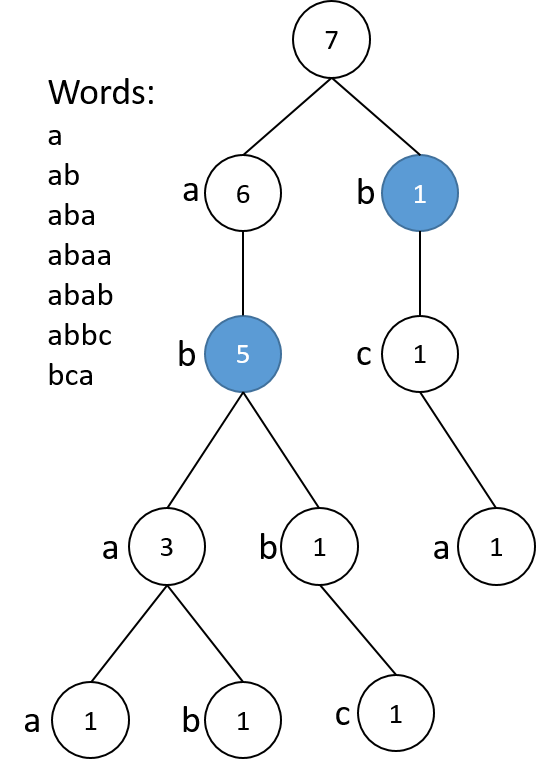题目大意

• 集合中任意前缀对应的单词数量小于等于5
• 对于集合中任意前缀`p``p`的扩展前缀不属于该集合

解题思路• 当节点数字小于等于5时，则表示该前缀是一个合法解
• 当节点为合法解时，以它为根的子树上其他节点都不再计入合法解

1. 对构建好的Trie树进行遍历
2. 若当前节点计数小于等于5，返回1
3. 若当前结点计数大于5，递归对所有子树进行遍历，并返回统计和

``````Traversal(root)
If (root.cnt <= 5)
Return 1
End If
total = 0
For i = 'a' .. 'z'
If root.chd[i] is not empty
total = total + Traversal(root.chd[i])
ENd If
End For
``````

• 如果a的子树有ca前缀，b的也有，是不是应该存在ca前缀，但是这个树却得不到

• 添加评论

``````#include<string>
#include <iomanip>
#include<fstream>
#include<set>
#include<queue>
#include<map>
//#include<unordered_set>
//#include<unordered_map>
//#include <sstream>
//#include "func.h"
//#include <list>
#include<stdio.h>
#include<iostream>
#include<string>
#include<memory.h>
#include<limits.h>
//#include<stack>
#include<vector>
#include <algorithm>
using namespace std;

struct TrieNode{
int count;
vector<TrieNode*> child;
TrieNode() :count(0), child(26,NULL){};
TrieNode(int x) :count(x), child(26,NULL){};
};
//插入单词
void InsertWord(char *a, int pos, TrieNode*p)
{
if (a[pos] == 0) return;//已经遍历完毕
else
{
//如果不存在，则新建儿子节点
if (p->child[a[pos] - 'a'] == NULL)
p->child[a[pos] - 'a'] = new TrieNode();
p->child[a[pos] - 'a']->count++;
InsertWord(a, pos + 1, p->child[a[pos] - 'a']);
}
}

//深度搜索
void dfs(int &ans, TrieNode*root)
{
//如果计数小于5，则不再遍历其儿子节点
if (root->count <= 5)
ans++;
else
{
//循环遍历儿子节点
for (int i = 0; i < 26; i++)
{
if (root->child[i])
{
dfs(ans, root->child[i]);
}
}
}
}
/*

*/
int main(void)
{
int n;
TrieNode *root = new TrieNode;
root->count = INT_MAX;
char *word = new char;
memset(word, 0, 10000);
cin >> n;

//输入单词
for (int i = 0; i < n; i++)
{
memset(word, 0, 10000);
scanf("%s", word);
InsertWord(word, 0, root);
}

//进行深度搜索
int ans = 0;
dfs(ans, root);
cout << ans << endl;

return  0;
}
``````
• 找到原因了，word分配的数组不够大，改为 char *word = new char;就可以了

• 添加评论
``````#include <iostream>
#include <string.h>
#include <string>

using namespace std;
typedef struct node trie;

typedef struct edge{
char ch;    //该边表示的字母
trie *child;   //指向的节点的指针
edge *next; //下一条边

}edgelist;
struct node{
int n;      //以该节点为根节点的树包括的单词数量
int sn;     //表示该节点是否是一个词尾
edgelist edges; //边的列表头结点
};

trie root;
trie * add_ch(char x, trie *rt)
{
edge * p = &(rt->edges);
while(p)
{
if(p->ch == x)
return p->child;
p = p->next;
}
p = &(rt->edges);
while(p->next) p = p->next;
p->next = new edge;
memset(p->next, 0, sizeof(edge));
p->next->ch = x;
p->next->child = new node;
memset(p->next->child, 0, sizeof(node));
return p->next->child;
}
{
trie *proot = &root;
for(int i=0; i<word.length(); ++i)
{
proot->n += 1;
}

proot->sn = 1;

}
int query2(trie * rt)
{
if(rt->n <=5)
return 1;
int sum = 0;
edge *p = rt->edges.next;
while(p)
{
sum += query2(p->child);
p = p->next;
}
return sum;
}
int main()
{
int n;
cin >>n;
root.n = n;
while(n--)
{
string word;
cin >> word;
}
int sum = 0;
edge *p = root.edges.next;
while(p)
{
sum += query2(p->child);
p = p->next;
}
cout << sum;
return 0;
}
``````

12 a ab abc abcde abcde abcba bcd bcde bcbbd bcac bee bbb

``````private static final int COUNT_LIMIT = 5;

public static void main(String[] args) {
String[] str = { "a", "ab", "aba", "abaa", "abab", "abbc", "bca" };
ShortestProperPrefix spp = new ShortestProperPrefix();
List<String> res = spp.shortestPrefix(Arrays.asList(str));
System.out.println(res);
}

// "" is a prefix; consider duplicated words not.
public List<String> shortestPrefix(List<String> words) {
List<String> res = new ArrayList<>();
TrieNode root = buildTrie(words);
preorderTraverse(res, "", root);
return res;
}

private void preorderTraverse(List<String> res, String string, TrieNode root) {
if (root == null) {
} else if (root.count <= COUNT_LIMIT) {
} else {
for (Map.Entry<Character, TrieNode> e : root.child.entrySet()) {
preorderTraverse(res, string + e.getKey(), e.getValue());
}
}
}

private TrieNode buildTrie(List<String> words) {
TrieNode root = new TrieNode();
return root;
}

private class TrieNode {
private int count;
private Map<Character, TrieNode> child;

TrieNode() {
this.count = 0;
this.child = new HashMap<>();
}

for (String e : s) {
}
}

}

private void add(String s, int i) {
count++;
if (i < s.length()) {
Character c = Character.valueOf(s.charAt(i));
if (child.get(c) == null) {
child.put(c, new TrieNode());
}